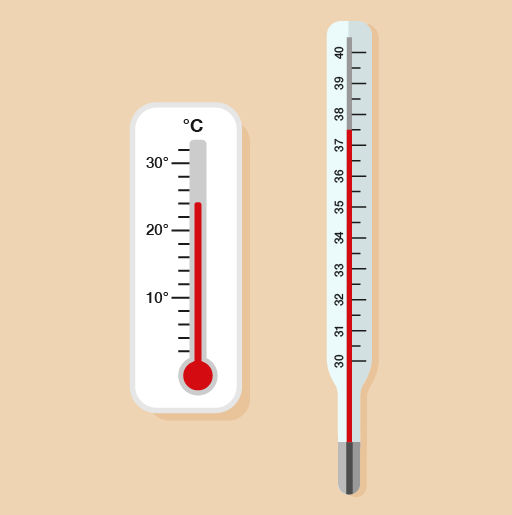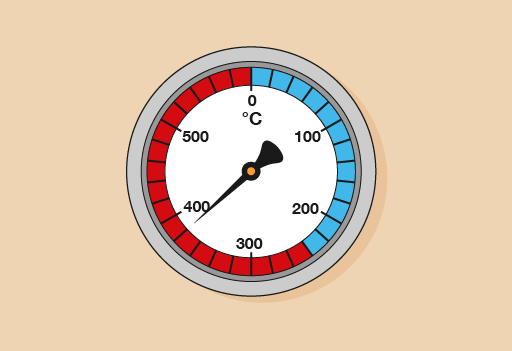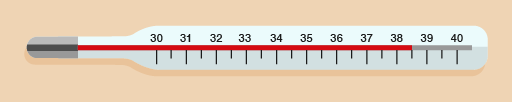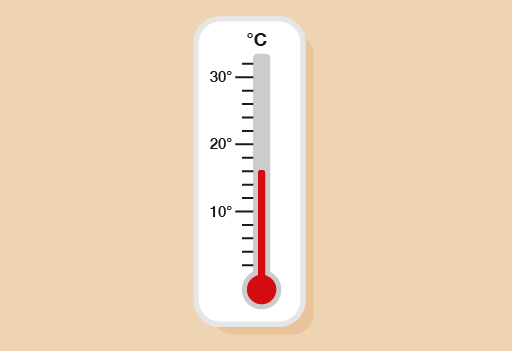Everyday maths 1 (Wales)

This free course is available to start right now. Review the full course description and key learning outcomes and create an account and enrol if you want a free statement of participation.

Free course

Everyday maths 1 (Wales)

Many things have to be stored or used in a particular temperature range to be safe. Temperature is measured with a thermometer.

Thermometers for different uses show different ranges of temperatures.

Take a look at the following example, which shows two types of thermometer.

What is the temperature shown on each thermometer below?Method

On the first thermometer, there are four divisions between 20 and 30, so the divisions mark every two degrees (22, 24, 26, 28). The reading is at the second mark after 20, so the temperature is 24°C.

On the second thermometer, the temperature is at the mark halfway between 37 and 38, so it’s 37.5°C.

What temperature do you think it is today? If you have a thermometer, check the temperature outside; if you don’t, you could use an online resource such as the BBC Weather pages [Tip: hold Ctrl and click a link to open it in a new tab. (Hide tip)] or your mobile phone to find the temperature near you.

Now try the following activity. Remember to check your answers once you have completed the questions.

What temperature is shown on each of these thermometers?

1.Figure 36 A thermometer
2.Figure 37 A thermometer
3.Figure 38 A thermometer

1. Each mark on the thermometer represents 20°C and the needle is at the mark below 400, so the temperature is 380°C.
2. The reading is on the mark halfway between 38°C and 39°C, so the temperature is 38.5°C.
3. Each mark represents 2°C, so the temperature is 16°C.
FSM_1_CYMRU